Courses

# Long Answer Type Questions- Areas of Parallelograms and Triangles Class 9 Notes | EduRev

## Class 9 Mathematics by Full Circle

Created by: Full Circle

## Class 9 : Long Answer Type Questions- Areas of Parallelograms and Triangles Class 9 Notes | EduRev

The document Long Answer Type Questions- Areas of Parallelograms and Triangles Class 9 Notes | EduRev is a part of the Class 9 Course Class 9 Mathematics by Full Circle.
All you need of Class 9 at this link: Class 9

Question 1. In a parallelogram ABCD, it is being given that AB = 12 cm and the altitude corresponding to the sides AB and AD are DL = 5 cm and BM = 8 cm respectively. Find AD.
Solution:
∵  Area of a parallelogram = base x height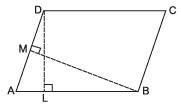∴ Area of parallelogram ABCD = AB x DL = 12 cm x 5 cm = 60 cm2 Again, area of parallelogram ABCD = AD x BM
∴  AD x BM = 60 cm2                    [∵ ar (parallelogram ABCD) = 60 cm2]
⇒ AD x 8 cm = 60 cm2                 [∵ BM = 8 cm (Given)]
= (15/2) cm = 7.5 cm
Thus, the required length of AD is 7.5 cm.

Question 2. Find the area of a trapezium whose parallel sides are 9 cm and 5 cm respectively and the distance between these sides is 8 cm.
Solution:
Let ABCD be a trapezium such that AD || BC. Let us join BD.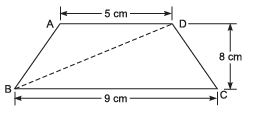∵ Area of a triangle = (1/2)x base x height
∴ Area of ΔABD = (1/2)x AD x height
= (1/2)x 5 cm x 8 cm = 20 cm2
ar (ΔBCD) = (1/2)x base x height
= (1/2)x BC x height
= (1/2)x 9 cm x 8 cm
= 36 cm
Now, ar (trapezium ABCD) = ar (D ABD) + ar (D BCD)
= 20 cm2 + 36 cm2
= 56 cm2

Question 3. In the adjoining figure, find the area of the trapezium ABCD.
Solution:
∵ DEC is a right triangle.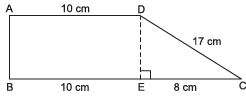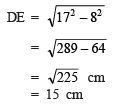∴ ar (ΔDEC) = (1/2)x base x height
= (1/2)x 8 x 15 cm2
= 60 cm2 ar (rectangle ABED) = length x breadth
= 10 cm x 15 cm = 150 cm2
Now, ar (trapezium ABCD) = ar (rectangle ABED) + ar (ΔDEC)
= 150 cm2 + 60 cm2 = 210 cm2
Thus, the required area of trapezium ABCD = 210 cm2.

Question 4. In the figure BD || CA, E is mid-point of CA and BD = (1/2) CA.
Prove that ar (
ΔABC) = 2 ar (ΔDBC)
Solution.
Join D and E ∵ BD = CE and BD || CE
∴ BCED is a parallelogram. ⇒ ΔDBC and ΔEBC are on the same base BC and between the same parallels,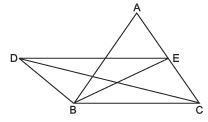∴ ar (ΔDBC) = ar (ΔEBC)                 ... (1)
In ΔABC, BE is a median,
∴ ar (ΔFBC) = (1/2)ar (ΔABC)
Now, ar (ΔABC) = ar (ΔEBC) + ar (ΔABE)
Also, ar (ΔABC) = 2 ar (ΔEBC)
∴ ar (ΔABC) = 2 ar (ΔDBC)

Question 5. In the adjoining figure, ABCD is a quadrilateral in which diagonal BD = 12 cm. If AL ⊥ BD and CM ⊥ BD such that AL = 6 cm and CM = 4 cm, find the area of quadrilateral ABCD.
Solution:
The diagonal BD divides the given quadrilateral into two triangles BDC and ABD.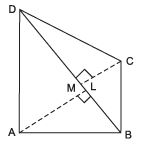Since, area of a triangle = (1/2)x base x altitude
∴ Area of ΔABD = (1/2)x BD x AL
= (1/2)x 12 cm  x 6 cm
= 36 cm2
Area of ΔBCD = (1/2)x base x altitude
=(1/2)x BD x CM
= (1/2)x 12 cm x 4 cm
= 24 cm2.
Now, ar (quadrilateral ABCD) = ar (ΔABD) + ar (ΔBCD)
⇒ ar (quadrilateral ABCD) = 36 cm2 + 24 cm2
= 60 cm2
Thus, the required area of quadrilateral ABCD
= 60 cm2.

Question 6. Find the area of the following quadrilateral.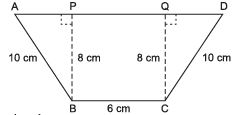Solution: ∵ APB is a right triangle.
∴ AB2 – BP= AP2
⇒ 102 – 8= AP2
⇒ 100 – 64 = AP
⇒ AP = 36 = 6 cm
Since, ar (rt ΔAPB) =(1/2)x base x altitude
∴ ar (ΔAPB) =(1/2)x 6 cm x 8 cm
= 24 cm2
Similarly, ar (rt ΔCQD) = 24 cm2 Area of rectangle BCQP
= length x breath = 6 cm x 8 cm = 48 cm2
= ar (rt ΔAPB) + ar (rectangle BCQP) + ar (rt ΔCQD)
= 24 cm2 + 48 cm2 + 24 cm2
= 96 cm2
Thus, the required area of the quadrilateral ABCD
= 96 cm2.

,

,

,

,

,

,

,

,

,

,

,

,

,

,

,

,

,

,

,

,

,

;